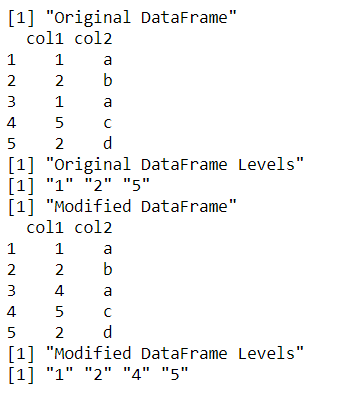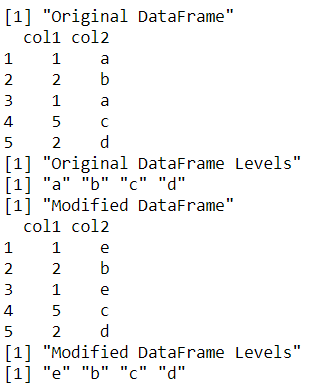Open in App
Not now

# Replace contents of factor column in R dataframe

• Last Updated : 12 Dec, 2021

In this article, we are going to see how to replace the content of the factor column in the dataframe in R Programming Language.

### Example 1: Replacing content of factor column

Initially, the factor column is converted to a character column using the explicit conversion of as.character() method in R. This converts the specified column to character type, which can contain duplicate element values, without all copies belonging to the same level.

This is followed by the replacement of the old cell value of this column with a new one. The column is then revered back again to factor type using as.factor() method.

The levels of the data frame column can be printed using the levels() method. If we display the levels before and after replacement, they will differ.

## R

 `# creating a data frame``data_frame <- ``data.frame``(col1 = ``factor``(``c``(1,2,1,5,2)),``                         ``col2 = ``factor``(``c``(``"a"``,``"b"``,``"a"``,``"c"``,``"d"``))``                         ``)``print``(``"Original DataFrame"``)``print ``(data_frame)` `print ``(``"Original DataFrame Levels"``)``print``(``levels``(data_frame\$col1))` `# replicating the data frame``data_temp <- data_frame` `# replacing the content of data frame``data_temp\$col1 <- ``as.character``(data_frame\$col1)``data_temp\$col1 <- 4``data_temp\$col1 <- ``as.factor``(data_temp\$col1)` `print``(``"Modified DataFrame"``)``print``(data_temp)` `print ``(``"Modified DataFrame Levels"``)``print``(``levels``(data_temp\$col1))`

Output:### Example 2: Replacing level of the factor column

The levels of the data frame can also be modified using the levels() method. The old level value can be compared using the data frame indexing method and then assigned a new value. All the occurrences of this level are changed to the new assigned level. The number of levels, in this case, remain same in number but change in value.

## R

 `# creating a data frame``data_frame <- ``data.frame``(col1 = ``factor``(``c``(1,2,1,5,2)),``                         ``col2 = ``factor``(``c``(``"a"``,``"b"``,``"a"``,``"c"``,``"d"``))``                         ``)``print``(``"Original DataFrame"``)``print ``(data_frame)` `print ``(``"Original DataFrame Levels"``)``print``(``levels``(data_frame\$col2))` `# replicating the data frame``data_temp <- data_frame` `# replacing the level of data frame``levels``(data_temp\$col2)[``levels``(data_temp\$col2) == ``"a"``] <- ``"e"``print``(``"Modified DataFrame"``)``print``(data_temp)` `print ``(``"Modified DataFrame Levels"``)``print``(``levels``(data_temp\$col2))`

Output:My Personal Notes arrow_drop_up